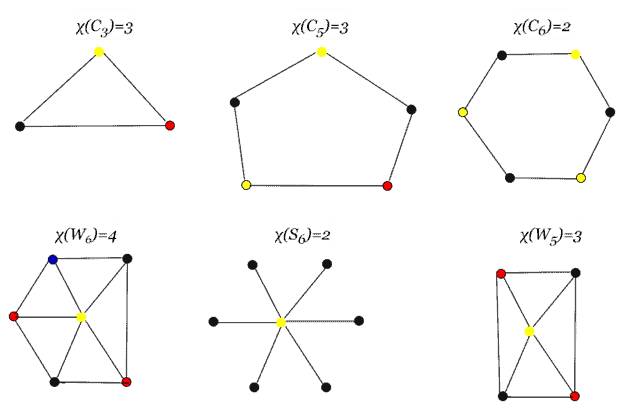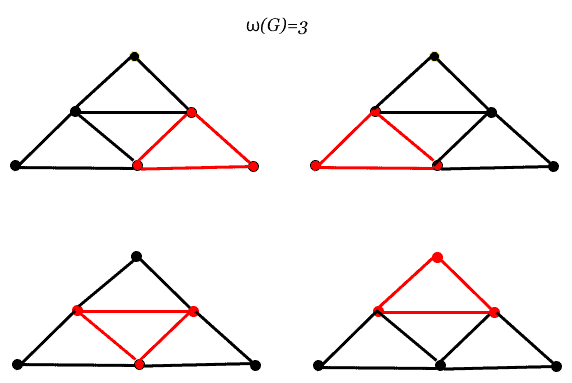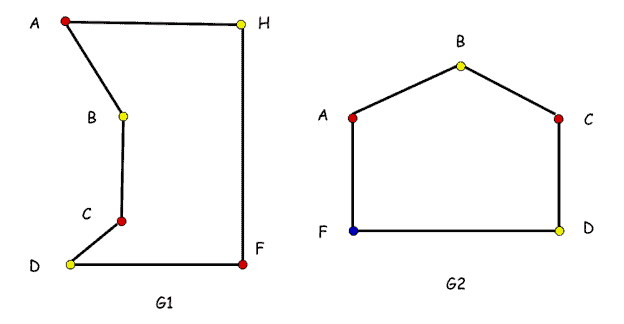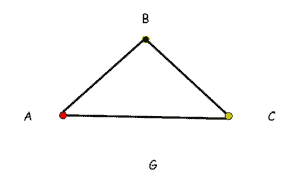## 1. Overview

In this tutorial, we’ll explore the definition of the perfect graph and its theorem in depth. Then, we’ll examine its mathematical implications and the key characteristics of perfect graphs. In addition, we’ll look at how perfect graphs are used in practice. Finally, we define the Strongly Perfect and the Weakly Perfect, which are used to classify perfect graphs.

## 2. What Exactly Is Graph Theory?

The study of graphs in both computer science and mathematics is known as graph theory. A graph is a mathematical structure employed to construct pairwise relationships between objects in a collection. In this context, a graph is a collection of nodes, also known as vertices, and the edges that connect them:The graph above demonstrates this:

• Graph• Vertices• Edges## 3. What Are Perfect Graphs?

A graphis said to be perfect if and only if each induced subgraph ofhas a clique number equal to its chromatic number, in other words,. In this context, a non-perfect graph is known as an imperfect graph. Let’s study these characteristics in the following subsections.

### 3.1. Chromatic Number of a Graph

The least amount of colors necessary to color the vertices of a graphso that no two adjacent nodes have the same color is its chromatic number.

The chromatic number of a graphis most frequently written as.

The graphs below show the minimal colorings and chromatic numbers for a selection of them:### 3.2. Clique Number of a Graph

A clique is a vertex-induced subgraph of a complete graph. A setis a clique of an graphif and only ifandand.

A graph G’s clique number, denoted, is the number of vertices in its maximum clique. It is equivalently the size of G’s largest clique or maximal clique.

As previously stated, a clique of a graphis a complete subgraph of. A clique is maximal if and only if it’s not a proper subgraph of another clique. A maximum clique of a graphis a clique with as many or more vertices as any other clique of.

The clique of a graph is illustrated in the graphs below, and the number of cliques for a single graph G is shown in red:### 3.3. The Definition of Perfect Graphs

A graphis perfect graph if for all,.

It means that the chromatic and clique number for each graph’s induced subgraphs must match for a graph to be considered perfect.

Let’s see some examples based on the following graphs:Since the clique numberin a graphequals the chromatic number, it is a perfect graph.and, soHowever, the chromatic numberfor graphis 4, and the clique numberis 3. As a result, graphis not a perfect graph.

Letbe a graph. A suitable coloring ofis a functionfromto some set, so thatwhenever. The chromatic number ofis the size of the smallest setfor which there exists a suitable coloring offromto.

We denote bythe largestclique’s size and bythe chromatic number of. It is true for every graphthat. It is said that a graph is perfect if, for each induced subgraphof,.

A perfect cycle with three vertices is depicted in the graph below:•Cycle with 3 vertices
•  Chromatic number• Clique numberA, B, and C are cliques in this graph, and the clique number is 3. Consequently,since. Accordingly, the clique number of graphis equal to the chromatic number of graph. As a result, thecycle graph is a perfect graph.

## 4. Strong Perfect Graph

A graph is strongly perfect if each induced subgraph H has a unique vertex set that satisfies each of H’s maximal cliques. Every strong perfect graph is perfect, but the opposite is not always true. The figure next presents a strong perfect graph:## 5. Weak Perfect Graph

A graph is weakly perfect if. This condition must hold for induced subgraphs to say a graph is perfect, but there is no requirement that it does. The clique number is, and the chromatic number is. Thus, in every situation, weak perfect graphs exist. The following example shows a weak perfect graph:## 5. Conclusions

This article covered perfect graphs, one of the most popular graph theories. At first, we discussed the perfect graph theorem. Then, to better understand the concepts, examples were used to explain the graphs’ chromatic and clique numbers. Finally, we presented both strong perfect graphs and weak perfect graphs. Readers will consequently have a thorough understanding of perfect graphs and their potential applications.